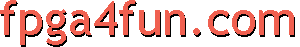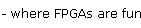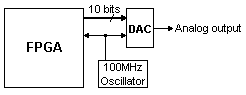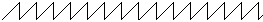## Direct Digital Synthesis 1 - Introduction

Let's see how easy an FPGA DSS implementation can be.

#### DAC

Ok, your new FPGA board has a fast DAC (digital-to-analog converter) analog output. Here's a possible board setup with a 10bit DAC running at 100MHz.At 100MHz, the FPGA provides a new 10bit value to the DAC every 10ns.
The DAC outputs an analog signal, and for periodic signals, the Nyquist limit says that speeds up to 50MHz can be achieved.

#### A simple DDS

A DDS is often used to generate periodic signals. For now, let's try something simple and generate a square wave.

```module SimpleDDS(DAC_clk, DAC_data);
input DAC_clk;
output [9:0] DAC_data;

// let's create a 16 bits free-running binary counter
reg [15:0] cnt;
always @(posedge DAC_clk) cnt <= cnt + 16'h1;

// and use it to generate the DAC signal output
wire cnt_tap = cnt;     // we take one bit out of the counter (here bit 7 = the 8th bit)
assign DAC_data = {10{cnt_tap}};   // and we duplicate it 10 times to create the 10-bits DAC value
// with the maximum possible amplitude
endmodule
```

We used the 8th bit of the counter to generate our output. With a counter clocked at 100MHz, the 8th bit toggles at a frequency of 100MHz/2^8=390KHz. So the DAC output is a 390KHz square signal.Now if we want a sawtooth wave, let's replace the last two lines of the code with this one:

```assign DAC_data = cnt[9:0];
```A triangular signal isn't difficult either.

```assign DAC_data = cnt ? ~cnt[9:0] : cnt[9:0];
```We created a DSS, all right. But a real-world DDS would allow us to:

• Create any shaped signal.
• Create any frequency signal.